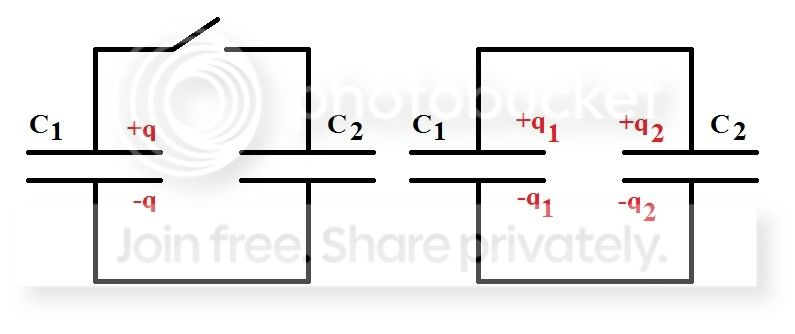# Why did the electric potential energy fall in this situation?

Suppose you have two capacitors configured as shown in the image below. One of them has charge $q$ and the other one is uncharged. Then, you close the switch, allowing charges to flow. After everything stabilizes, we now have another charge distribution, as shown in the image.We know that

$$\frac{q_1}{C_1} = \frac{q_2}{C_2}$$ $$q_1 + q_2 = q$$

Let's calculate the electric potential energy stored before closing the switch (let's call that $U_0$) and after closing the switch (let's call that $U_f$).

I calculated those and found that

$$U_0 = \frac{q^2}{2C_1}$$ $$U_f = \frac{q^2}{2(C_1+C_2)}$$

This means that the electric potential energy was lowered. What happened to the rest of the energy?

At first, I thought this difference of energy became kinetic energy for the charges to move around. But, in the end of everything, those charges stop. The total change of kinetic energy is zero!! What happened? What am I missing?

# The rest of the energy is basically emitted as heat energy.

Why?

You have two capacitors in the circuit, and the connecting wires offer negligible resistance.

Hence, when electrons flow from the charged capacitor to the uncharged one, the electrons basically face no resistance, and they collide with high speed with the uncharged capacitor. This collision leads to loss of energy in the form of heat energy.

After the first few electrons reach the capacitor, they start repelling the other electrons coming with high speeds, and hence, the loss of energy reduces as the capacitor is being charged.

Try this practically. You'll find the uncharged capacitor has become hot, due to this.

• Excellent. Thank you very much. By the way, your answer created another question in my mind, I'll create a separate question for it later. Thanks! – Pedro A May 22 '15 at 14:08